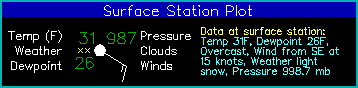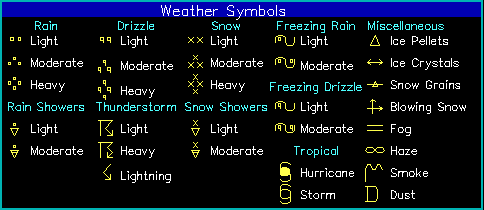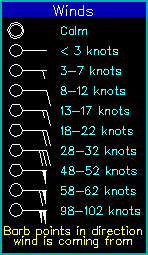Here is a graphic showing a breakdown of the plots.

### Surface Data Plot

The surface data plot gives the following information:Data Description temperature (F) plotted upper left present weather symbol plotted center left (see below) dewpoint (F) plotted lower left pressure (.1 mb-coded) plotted upper right as last 3 digits (987=998.7mb, 024=1002.4mb) cloud cover center circle. white fill indicates % cloud coverage (see below) winds wind barb (see below)

### Weather Symbols